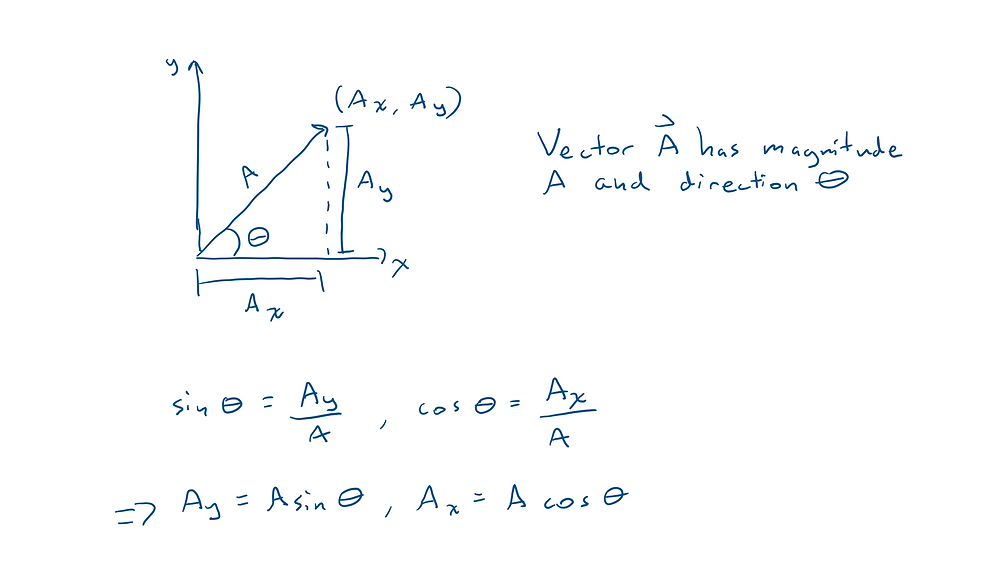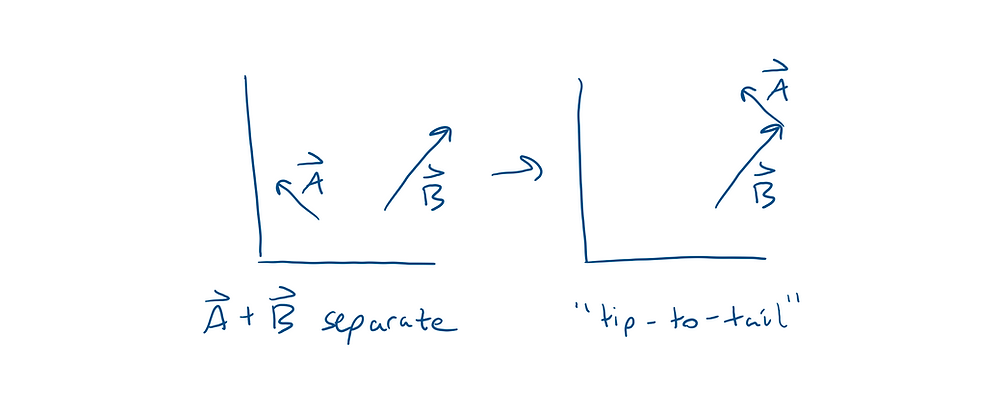# Vectors

A vector is just an arrow from the origin to a point. It has magnitude (how big it is) and direction (which way it’s pointing). A variable will have an arrow above it if it’s a vector!

Vectors can be in any number of dimensions,but usually they are in 2D or 3D.

If I have 2 vectorsI can add them component-wise, meaning you add the x-components together, the y-components together, and the z-components together:To subtract two vectors, just do the same thing but with a minus sign:If I know a 3D vector’s magnitude and direction, and I want to find its components, I can just use trig:* Make sure to find A_x and A_y with SOH CAH TOA each time because A_y doesn’t always correspond with sin θ, and A_x doesn’t always correspond with cos θ *

### Tip-to-Tail Method

When you want to add or subtract two vectors in a picture use the tip-to-tail method. Here are the rules:

1) You can move any vector anywhere you want as long as you don’t change its magnitude (length) or its direction (angle)2) Place the tip of one vector on the tail of the other (it doesn’t matter which tip goes on which tail)3) Now draw an arrow pointing from the tail of the first vector to the tip of the second, and you’ve added your two vectors together!4) If you want to subtract one vector form another, all you have to do is flip the tip and tail of the vector you want to subtract, and then do tip-to-tail## Alex Koek

December 10, 2019

View Profile

#### Get in Touch

Thanks! We'll reach out shortly.